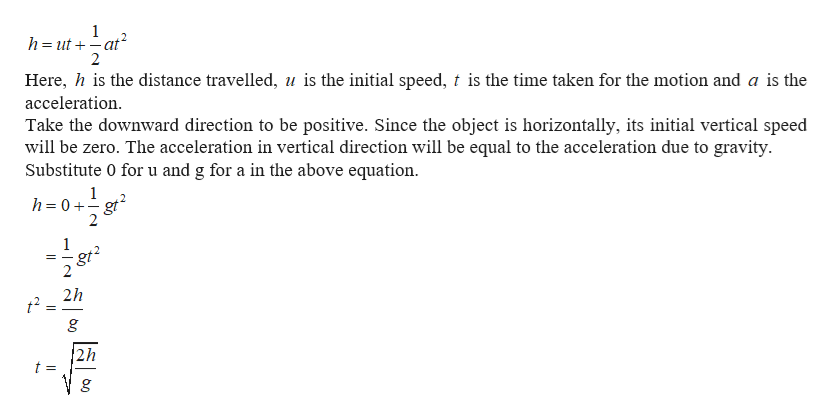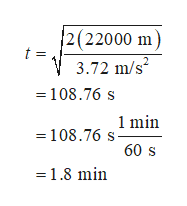# The highest mountain on Mars is Olympus Mons, rising 22 000 meters above the Martian surface. If we were to throw an object horizontally off the mountain top, how long would it take to reach the surface? (Ignore atmospheric drag forces and use g Mars = 3.72 m/s 2.) a.2.4 minutes b.0.79 minute c.1.8 minutes d.3.0 minutes

Question

The highest mountain on Mars is Olympus Mons, rising 22 000 meters above the Martian surface. If we were to throw an object horizontally off the mountain top, how long would it take to reach the surface? (Ignore atmospheric drag forces and use g Mars = 3.72 m/s 2.)

a.
2.4 minutes

b.
0.79 minute

c.
1.8 minutes

d.
3.0 minutes

check_circle

Step 1

The equation of motion is given byhelp_outlineImage Transcriptionclose1 h ut-at 2 Here, h is the distance travelled, u is the initial speed, t is the time taken for the motion and a is the acceleration Take the downward direction to be positive. Since the object is horizontally, its initial vertical speed will be zero. The acceleration in vertical direction will be equal to the acceleration due to gravity Substitute 0 for u and g for a in the above equation 1 h= 0+gt 2 2 1 gt2 2h 2h t = fullscreen
Step 2

Substitute the numerical values...help_outlineImage Transcriptionclose2(22000 m t = 3.72 m/s 108.76 s 108.76 smin 60 s 1.8 min fullscreen

### Want to see the full answer?

See Solution

#### Want to see this answer and more?

Solutions are written by subject experts who are available 24/7. Questions are typically answered within 1 hour.*

See Solution
*Response times may vary by subject and question.
Tagged in

### Newtons Laws of Motion